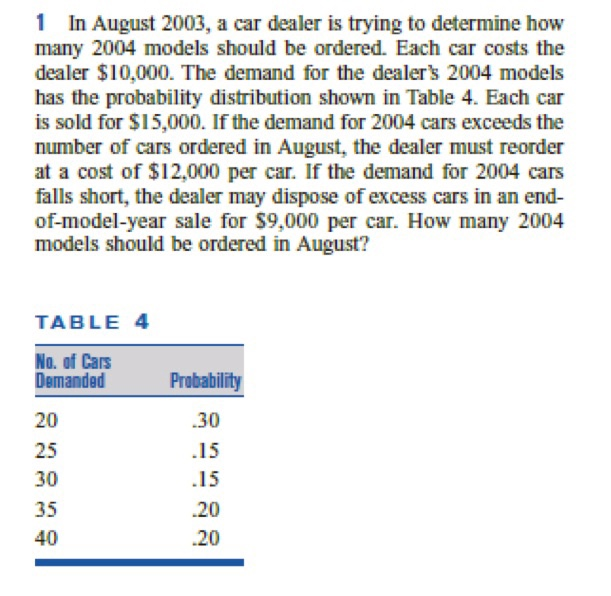# Question Solved1 Answer1 In August 2003, a car dealer is trying to determine how many 2004 models should be ordered. Each car costs the dealer $10,000. The demand for the dealer's 2004 models has the probability distribution shown in Table 4. Each car is sold for$15,000. If the demand for 2004 cars exceeds the number of cars ordered in August, the dealer must reorder at a cost of $12,000 per car. If the demand for 2004 cars falls short, the dealer may dispose of excess cars in an end- of-model-year sale for$9,000 per car. How many 2004 models should be ordered in August? TABLE4 No. of Cars Demanded Probability 20 25 30 35 40 .30 .15 .15 .20 .206OLRNU The Asker · Probability and StatisticsTranscribed Image Text: 1 In August 2003, a car dealer is trying to determine how many 2004 models should be ordered. Each car costs the dealer $10,000. The demand for the dealer's 2004 models has the probability distribution shown in Table 4. Each car is sold for$15,000. If the demand for 2004 cars exceeds the number of cars ordered in August, the dealer must reorder at a cost of $12,000 per car. If the demand for 2004 cars falls short, the dealer may dispose of excess cars in an end- of-model-year sale for$9,000 per car. How many 2004 models should be ordered in August? TABLE4 No. of Cars Demanded Probability 20 25 30 35 40 .30 .15 .15 .20 .20
More
Transcribed Image Text: 1 In August 2003, a car dealer is trying to determine how many 2004 models should be ordered. Each car costs the dealer $10,000. The demand for the dealer's 2004 models has the probability distribution shown in Table 4. Each car is sold for$15,000. If the demand for 2004 cars exceeds the number of cars ordered in August, the dealer must reorder at a cost of $12,000 per car. If the demand for 2004 cars falls short, the dealer may dispose of excess cars in an end- of-model-year sale for$9,000 per car. How many 2004 models should be ordered in August? TABLE4 No. of Cars Demanded Probability 20 25 30 35 40 .30 .15 .15 .20 .20Answer: Given Data Let , q = Number of 2004 model cars ordered in August d = Number of 2004 model cars demanded after August Now determine the smallest value of q for which&#160;E(q+1)-E(q) >= 0&#160; To calculate&#160;E(q+1)-E(q)&#160;, consider two possibilities: Case 1 : If&#160;d <= q&#160; then in this case , ordering q + 1&#160; units instead of q units which cause to be overstocked by one more unit . The probability that Case 1 will occur is simply&#160;P(D <= q)&#160;,&#160; where D is the random variable representing demand. Case 2 : If&#160;d >= q+1&#160;&#160;then in this case , ordering q + 1&#160; units instead of q units enables to be short one less unit . The probability that&#160; Case 2 will occur is&#160;P(D >= q+1)=1-P(D <= q) Now check conditions written above for the give problem. If&#160;d <= q&#160;, the costs shown in table given below are incurred t tt tttComputation of Total Cost if&#160;d <= q ttt&#160; tt tt ttt&#160; tttCost tt tt tttBuy q cars at $10000/car ttt10000q tt tt tttSell d cars at$15000/car ttt-15000d tt tt tttDispose of excess q-d cars at \$9000/car ttt-9000(q-d) tt tt tttTotal cost ttt10000q-15000d-9000(q-d) tt t Hence total cost for case&#160;d <= q&#160;is , 10000 q-15000 d-9000(q-d)=10000 q-15000 d-9000 q+9000 d quad rarr(1)&#160; &#160; &#160; &#160; &#160; &#160; &#160; &#160; &#160; &#160; &#160; &#160; &#160; &#160; &#160; &#160; &#160; &#160; &#160; &#160; &#160; &#160; &#160; &#160; &#160; &#160; &#160; &#160; &#160; &#160; &#160; &#160; &#160;=1000 q-6000 d Compare equation (1) with equation , c(d,q) =&#160;c_(0)q&#160;+ (terms not involving q) Hencew&#160;C_(0)&#160; is the per - unit cost of being overstocked . Thus get the value&#160;C_(0)&#160;= 1000 If&#160;d >= q+1&#160; , the costs shown in table given below are incurred t tt tttComputation of Total Cost if&#160;d >= q+1 ttt&#160; tt tt ttt&#160; tttCost tt tt t ... See the full answer